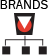Create Config
Edit Name Description Configiration Type Type

# Calculators

## Technical Reference

NOTE:      Enter values in shaded fields below in the indicated units of measure.

For 2-part reeving, the diagonal spacing values (Sp & Sb) entered below would simply be the spacing at each associated single sheave.

The results of this calculator are approximations only. The calculator is not intended for design.

Resulting plotted points landing within a wire rope class band indicate uncertain stability for that rope class. Points below the band will most likely be stable. Points above the band will most likely be unstable.

Block Stability Calculator
Block Stability Calculator
L =
Sp =
Sb =
Save
P =
S =
Dp =
Db =
Dave =
d =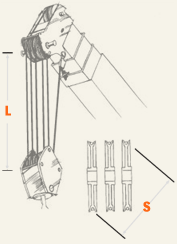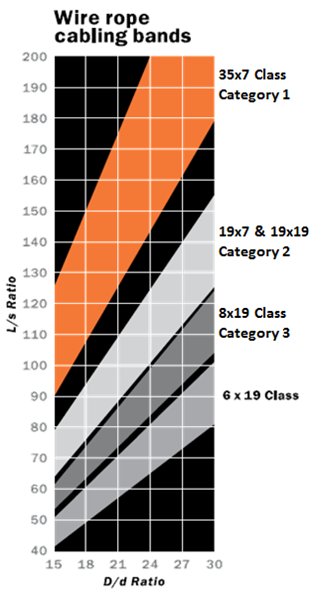## Metallic Area/Elastic Stretch Calculator

This calculates the metallic cross-sectional area in a rope and then uses this information to calculate elastic stretch in a rope that occurs under load.  Click here to view our Metallic Area/Elastic Stretch Calculator.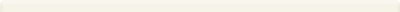Metallic Area / Elastic Stretch Calculator
Approximate Metallic Areas
Wire Rope Approximate Elastic Stretch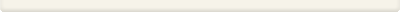## Drum Capacity Calculator

This calculates how much rope of a given diameter can be spooled onto a reel of a given size.  Click here to view our Drum Capacity Calculator.Drum Capacity Calculator
Approximate Drum Capacity

1.
2.
3.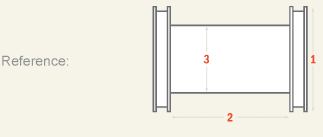## Metallic Area/Elastic Stretch Calculator

This calculates the metallic cross-sectional area in a rope and then uses this information to calculate elastic stretch in a rope that occurs under load.  Click here to view our Metallic Area/Elastic Stretch Calculator.Metallic Area/Elastic Stretch Calculator
Approximate Metallic Areas
/
TUF-STRAND
Approximate Elastic Stretch
Wire Rope
Approximate Elastic Stretch

## Block Stability Calculator

This determines if the reeving system in a hoist block will be stable (no block twisting) or unstable (block twisting will occur).  Click here to view our Block Stability Calculator.

NOTE:      Enter values in shaded fields below in the indicated units of measure.

For 2-part reeving, the diagonal spacing values (Sp & Sb) entered below would simply be the spacing at each associated single sheave.

The results of this calculator are approximations only. The calculator is not intended for design.

Resulting plotted points landing within a wire rope class band indicate uncertain stability for that rope class. Points below the band will most likely be stable. Points above the band will most likely be unstable.

Block Stability Calculator
Block Stability Calculator
L =
Sp =
Sb =
Save
P =
S =
Dp =
Db =
Dave =
d =## Choker Rated Capacity Calculator

This calculates the rated capacity for a sling used in a choker hitch.  Click here to view our Choker Rated Capacity Calculator.Choker Rated Capacity Calculator
Choker Rated Capacity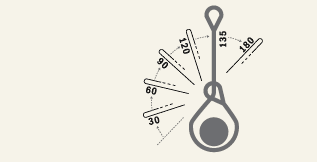Angle / Leg Length (2 leg slings only)

Enter any two known values: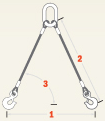1.
2.
3.## Drum Capacity Calculator

This calculates how much rope of a given diameter can be spooled onto a reel of a given size.  Click here to view our Drum Capacity Calculator.Drum Capacity Calculator
Approximate Drum Capacity
1.   /
2.   /
3.   /
/## Metric Conversion for Standard Rope Diameters

This shows the metric size equivalent to imperial rope diameters.  Click here to view our Metric Conversion for Standard Rope Diameters.Metric Conversion for Standard Rope Diameters
/
/
=
"Soft" Metric Conversion for Standard Rope Diameters
=## Sling Calculator

This calculates the capacity of a wire rope sling based on the type of rope used in the sling and the angles at which the sling is used.  Click here to view our Sling Calculator.Sling Calculators
Sling Calculators
Angle / Leg Length (2 leg slings only)

Enter any two known values:1.
2.
3.